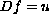Electron. J. Diff. Eqns., Vol. 2000(2000), No. 59, pp. 1-8.

### Differential operators on equivariant vector bundles over symmetric spaces Anton Deitmar

Abstract:
Generalizing the algebra of motion-invariant differential operators on a symmetric space we study invariant operators on equivariant vector bundles. We show that the eigenequation is equivalent to the corresponding eigenequation with respect to the larger algebra of all invariant operators. We compute the possible eigencharacters and show that for invariant integral operators the eigencharacter is given by the Abel transform. We show that sufficiently regular operators are surjective, i.e. that equations of the formare solvable for all.Anton Deitmar School of Mathematical Sciences University of Exeter Laver Building, North Park Road Exeter, EX4 4QE Devon, UK email: A.H.J.Deitmar@exeter.ac.uk